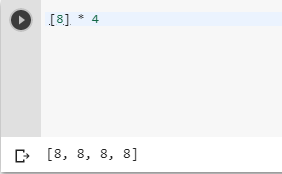2 views
in Python

I know a list comprehension will do this, but I was wondering if there is an even shorter (and more Pythonic?) approach.

I want to create a series of lists, all of the varying length. Each list will contain the same element e, repeated n times (where n = length of the list). How do I create the lists, without doing

[e for number in xrange(n)]

for each list?

by (106k points)

To create a list of Single Item Repeated n Times in Python, you can use the following piece of code:-

>>>[e] * n

An important note is if e is an empty list you get a list with n references to the same list, not n independent empty lists.

Following is the example of the above explanation -

Where e=8 and n=4+1 vote